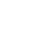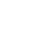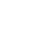# Euclid

### Early LifeEuclid of Alexandria (often confused with the Greek philosopher Euclid of Megara) was an ancient Greek Mathematician. Although there have been many attempts to guess the dates of his birth and death, we don’t know these for sure. However, we can say that he lived in around 300 BC. We also have a small amount of additional information from other Arabic sources about Euclid’s early life, but these are not thought to be reliable. The Arabian authors claim that Euclid was the son of a man called Naucrates. They also suggest that he was born in Tyre. Historians have suggested that this information is nothing more than a creative invention. Some scholars have even suggested that the lack of information on this hugely important figure may reflect the fact the Euclid was not an actual person, but a group of mathematical experts who used the name to collectively describe themselves. However, there is no real evidence to confirm this theory.

The Greek Philosopher Proclus, 410-485 AD, wrote his Commentary on the First Book of Euclid’s Elements and ittells us that Euclid taught in Alexandria. It is Proclus who reminds us that there was, in fact, a rich history of geometry before Euclid compiled his major work.

Proclus tells us about Euclid’s role as a collector of theorems and about his role in providing proof that those theorems were universally valid. However, even Proclus’ work was based on earlier commentaries so our knowledge about Euclid from this most important source still has its limitations.

Euclid is often seen as the “father of geometry”. He wrote several books but the most famous of these is The Elements which is one of the most important geometry and mathematics books that has ever been written. Euclid is among the most significant mathematicians in history, influencing theorists from ancient times to the present day.

### Existing Mathematics

The content of The Elements was not simply the product of one thinker. Mathematicians had been dealing with many of ideas in the book for hundreds of years, from arithmetic to the use of maths for practical purposes, such as trade and the construction of cities.

The Egyptians knew how to obtain approximate diameters and were very close to working out “pi” (ratio of a circle’s circumference to its diameter). In addition to circles, they also understood triangles and right angles.

Yet mathematics had become increasingly sophisticated in ancient Greece. Euclid took ideas from mathematicians, such as Pythagoras, Hippocrates,Theudius, Theaetetus and Eudoxus.

What Euclid did was to gather existing ideas in mathematics and create a standardised system of laws that would be applicable in any case. The idea that you could prove a general principle to be applicable to a specific case is known as deductive reasoning.

For instance, Euclid wanted to show why the square on the larger side of a triangle is equivalent to the sum of the two angles on the smaller side. The Greeks were very interested in logical thinking and systems and laws – structured ways of life that followed specific patterns.

### The Book’s Journey through Time

Euclid’s book has stood the test of time and has been the most important textbook on geometry for more than two centuries. It was used as the mathematical basis of a range of disciplines in ancient Rome and through the middle ages.

There are a number of different versions of the text, including an Arabic, Latin and a Greek variant and there are slight differences between these different translations of Euclid’s original work. Theon of Alexandria was a mathematician who lived in the 4th century AD and he edited Euclid’s original text; his additions were fairly limited.

In fact, his was the sole Greek version of the Elements available until an earlier edition was found in the Vatican during the 19th century. Theon attempted to make the text more accessible for his own pupils and a wider audience.

The Arabic version developed around the 8th century AD, under Harun Al Rashid the Caliph who presided over a period of intellectual and cultural growth in the Islamic world. He ordered for it, among other texts, to be obtained in Byzantium and brought back to Baghdad.

This was a time when interest in translating Greek texts led to the appearance of The Elements in that language. This was important as the translation into Arabic helped to preserve the ideas which eventually made their way to the present day.

Following the invention of the printing press in 1482, the book appeared in many different languages and is often seen as the most read book after the bible in the Western world.

### The Elements

The work is made up out of 13 books and these are broken up into three constituent parts covering plane geometry, arithmetic and solid geometry. The book begins with some basic definitions to help the reader make sense of what follows. Indeed, the entire book is a series of logical layers built on top of each other gradually getting more complex.

### The Definitions

The definitions include geometrical axioms (known as postulates). An axiom is something that we accept to be true. These appear to be extremely obvious to the modern reader, but these ideas were not always taken for granted:

It is possible to draw a straight line between two points

A straight line can be extended continuously in either direction

It is possible to describe and create a circle with any radius if there is an endpoint in the centre

All right angles measure the same at 90 degrees so they are congruent

Euclid begins with the idea that if you have two lines and then cross those two lines with another line you get two inner angles. Euclid believed that if those angles did not add up to 180 degrees then the two lines would eventually cross over one another.

### Part One – Plane Geometry

Pythagoras’ theorem was based on the idea of building squares on each side of a right triangle. He discovered that the area of the square drawn on the side of the hypotenuse is the same as the combined area of the squares on the remaining sides.

So as with many of the theories in Euclid’s The Elements, Euclid was not responsible for Pythagoras’ original idea as such. Nonetheless, he played a vital role in proving the theory by means of sophisticated dissections of Pythagoras’s original shape, showing that the different parts were equal to one another, even from different perspectives.

It was very much in the Greek tradition to prove that such theories were true and universally applicable. Indeed, books 1-4 are all preoccupied with similar plane geometry.

Book two deals with lines and ratios, book three looks at the structure of circles and book four examines how polygons, such as the pentagon, are structured. Book 5 looks at proportion and this is subsequently applied to the geometry featured in Book 6.

### Part two – about numbers

Books 7, 8 and 9 examine the fundamental ideas of arithmetic and convey some of the basic understanding of what numbers are. One of the most important conclusions of Euclid’s book concerns the role of numbers and how they work.

Euclid’s so-called Fundamental Theorem of Arithmetic suggests that every positive whole number that is more than one is either prime (a prime number can only be divided by itself or by 1) or can be made by multiplying different combinations of prime numbers.

So, for instance, if we multiply 2 x 11 (or 11 X 2), we get 22. If we multiply 3 x 7, we get 21. Only these particular combinations of prime numbers will produce these specific numbers. So Euclid reached the important conclusion that any number can be reached through a unique combination of prime numbers

Euclid was the first mathematician to prove the idea that there are infinitely many prime numbers: 2, 3, 5, 7, 11,13, 17, 19 and so on. This proof, which is something that is taken for granted today, is ingenious.

As the list of primes is finite, it is possible to multiply prime numbers together then add one. So, for example, 2 x 3 x 7 = 42. 42 is not a prime number as it can be divided by 7 and not just by itself or 1.

However, if we simply add 1, we get 43 which is a prime number because it is not divisible and it becomes a new prime number. This apparently straightforward discovery was absolutely vital for the development of number theory.

### Part Three – Solid Geometry

The final three books explain the principles of solid geometry. They show how the so-called Platonic solids are constructed and classified. The platonic solids are named after the ancient Greek philosopher Plato who wrote about them extensively.

Books 11 and 12 deal with the basic aspects of solid geometry, as well as areas. Platonic solids involve what we might describe as a geometry that is three dimensional. Euclid discusses lines and planes and other matters, such as the volumes of solids, including cylinders, spheres, pyramids and cones. He also delves into other important calculations, including the area of a circle.

Book 13 is especially important for the mathematician as it discusses the five solids. They are the tetrahedron (which is a triangular pyramid with four faces), cube (hexahedron with six faces), octahedron (eight faces), dodecahedron (with twelve faces) and icosahedrons (twenty faces).

This book shows how it is possible to construct these solids. For instance, Euclid explains that if we put four triangles together at a vertex (this means the point where two or more lines meet) then we can create a pyramid with a square base.

From here we can merge two such pyramids at their bases to produce an entirely symmetrical ensemble consisting of eight triangular faces. This is how an octahedron is formed.

Euclid reaches some fascinating conclusions in this part of his book. He suggests that if we draw a pentagon (with sides that are proportionate and equal) inside a circle, the side of the pentagon is exactly the same in square to a hexagon or even a decagon if you were to draw them in the very same circle.

This means that the lengths of the sides of a pentagon, hexagon or a decagon are all the same as the lengths of the sides of a right-angled triangle.

Another important conclusion of Euclid’s work is that the only possible regular solids that we can have are the tetrahedron, cube, octahedron, dodecahedron and icosahedrons. He proved that there are only five possibilities; there cannot be a sixth shape.

### Legacy

Euclid’s Elements have without any doubt had an enormous impact on human civilisation. His book is still respected as a basis for teaching children certain aspects of mathematics to this day. One of his main legacies is the idea that human beings can achieve absolute certainty in one form or another.

His idea of building upon axioms or given truths influenced great scientists, such as Issac Newton and philosophers, including Descartes. Our view of the world is still dominated by the possibility of discovering regular patterns and laws.

This does not mean that Euclid’s ideas have not been challenged in any way. Non-Euclidian alternatives have been suggested by a range of different thinkers. They suggest that one of the main problems of Euclid’s thinking is that it is based on a two-dimensional, flat world and they argue that not every object fits into the neat and tidy Euclidean picture of the world.

Therefore, they contend that much of Euclid’s system is not universally applicable in the way he thought. Nevertheless, it is still dominant and The Elementsremains one of the most important mathematical works.

•History
•Science
•Geography
•Biography
•US History